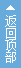# 传奇私服土城公告牌脚本实例

2018-01-23 00:31 | 作者：传奇私服发布网| 来源：新开传奇网站 | 阅读：次 |
[@main](2431,800,700,3,1,1,1,582,2,100,100) {f.3,x.132,y.102,b.0,i.2471,t.100=img} {f.3,x.132,y.182,b.0,i.2471,t.100=img} {f.3,x.132,y.262,b.0,i.2471,t.100=img} {f.3,x.323,y.102,b.0,i.2471,t.100=img} {f.3,x.323,y.182,b.0,i.2471,t.100=img} {
 [@main](2431,800,700,3,1,1,1,582,2,100,100) <{f.3,x.132,y.102,b.0,i.2471,t.100=img}> <{f.3,x.132,y.182,b.0,i.2471,t.100=img}> <{f.3,x.132,y.262,b.0,i.2471,t.100=img}> <{f.3,x.323,y.102,b.0,i.2471,t.100=img}> <{f.3,x.323,y.182,b.0,i.2471,t.100=img}> <{f.3,x.323,y.262,b.0,i.2471,t.100=img}> 　　　　　　　　　　　　　　　　　<公            告            牌>\ 　\ 　　　　　　　　　在本服务器最新活动都将会在这里公布，请注意查看这里公布的信息。\ \ \ \ \ 　　　　　　　　　　　　　　　　　　　　<荒野灵兽{【活动】=FCO=250}/@活动公告_荒野灵兽>\ \ \ \ \ 　　　　　　　　　　　　<天赐宝箱{【活动】=FCO=250}/@活动公告_天赐宝箱>　　　　　　　　<天下第一{【奖励】=FCO=250}/@活动公告_天下第一>\ \ \ \ \ 　　　　　　　　　　　　<攻沙奖励{【奖励】=FCO=250}/@活动公告_攻沙奖励>　　　　　　　　<结束对话{【关闭】=FCO=250}/@Exit>\ \ \ \ 　　　　　　　　　　　　　{当前时间:=FCO=1}{<\$DATETIME>=FCO=251}\   [@活动公告_攻沙奖励](2431,800,700,3,1,1,1,582,2,100,100) <{f.3,x.190,y.-5,b.0,i.2268,t.100=img}> <{f.3,x.156,y.293,b.0,i.2465,t.100=img}> <{f.3,x.300,y.293,b.0,i.2465,t.100=img}>\ \ \ \ \ 　　　　　　　　{活动名称：=FCO=243}攻沙奖励\ 　　　　　　　　{活动时间：=FCO=243}首次沙巴克攻城结束后\ 　　　　　　　　{活 动 一：=FCO=243}系统会在{[\$沙巴克首次攻城时间_年]年[\$沙巴克首次攻城时间_月]月[\$沙巴克首次攻城时间_日]日[\$沙巴克首次攻城时间_时]时=FCO=251}自动组织所有行会统一攻城战\ 　　　　　　　　　　　　争，到时将产生第一任沙巴克城主。首任沙巴克城主将得到\ 　　　　　　　　　　　　系统提供[\$元宝名称]奖励。{(首次攻城=FCO=250}{结束后沙城主可自己在沙巴克=FCO=250}\ 　　　　　　　　　　　　　　　　　　　　　　　　　　{官员那里，自助领取奖励！)=FCO=250}\ 　　　　　　　　{活动奖励：=FCO=243}{[\$首任城主元宝奖励数量]=FCO=253}{[\$元宝名称]=FCO=253}\ \ 　　　　　　　　{活 动 二：=FCO=243}在首次攻城战结束后，每次玩家自助申请攻城战，战争结束\ 　　　　　　　　　　　　 后，获胜方照样可以获得[\$元宝名称]奖励。{(如果没有发生战争，现=FCO=250}\ 　　　　　　　　　　　　　　　　　　　　　　　　　　　{任沙城主也无法领取奖励！)=FCO=250}\ 　　　　　　　　{活动奖励：=FCO=243}{[\$攻城战争结束奖励数量]=FCO=253}{[\$元宝名称]=FCO=253}\ \ \   　　　　　　　　　　　　　　　<返回上页/@main>　　　　　　　　<结束对话/@Exit>\ \ 　　　　　　　　　　　 {当前时间:=FCO=1}{<\$DATETIME>=FCO=251}\       [@活动公告_天下第一](2431,800,700,3,1,1,1,582,2,100,100) <{f.3,x.200,y.-10,b.0,i.2265,t.100=img}> <{f.3,x.170,y.60,b.0,i.2249,t.100=img}> <{f.3,x.156,y.293,b.0,i.2465,t.100=img}> <{f.3,x.300,y.293,b.0,i.2465,t.100=img}>\ \ \ \ \ \ \ 　　　　　　　　{活动名称：=FCO=243}天下第一\ 　　　　　　　　{活动时间：=FCO=243}[\$沙巴克首次攻城时间_年]年[\$沙巴克首次攻城时间_月]月[\$沙巴克首次攻城时间_日]日13点之前\ \ 　　　　　　　　{活动内容：=FCO=243}在盟重省安全区内有四尊雕像，请在活动时间结束之前，自己\ \ 　　　　　　　　　　去申请天下第一。在活动结束后，系统会记录当前天下第一名单。\ \ 　　　　　　　　　　　　　　　　玩家可自行到对应的雕像处领取奖励！\ \ 　　　　　　　　{活动奖励：=FCO=243}[\$天下第一元宝奖励数量][\$元宝名称]\ \ \ 　　　　　　　　　　　　　　　<返回上页/@main>　　　　　　　　<结束对话/@Exit>\ \     [@活动公告_守沙奖励] {活动名称：=FCO=243}守沙奖励\ {活动时间：=FCO=243}每天22:10-23:59之间\ {活动内容：=FCO=243}从攻下沙巴克之日起开始，每\ 　　　　　守住沙巴克不被其它行会占领\ 　　　　　1天，{守城时间=FCO=251}将自动增加1天，\ 　　　　　跟据守城的天数，将给予不同\ 　　　　　数量的元宝奖励。\ 　　　　　{已守城天数　　奖励元宝数量=FCO=243}\ 　　　　　　{   3天　　　　100元宝=FCO=242}\ 　　　　　　{   6天　　　　300元宝=FCO=242}\ 　　　　　　{   9天　　　　600元宝=FCO=242}\ 　　　　　　{  12天　　　 1000元宝=FCO=242}\ 　　　　　　{  15天　　　 1500元宝=FCO=242}\ 　　　　　{不过只要一领取奖励，守城天=FCO=254}\ 　　　　　{数将自动初始化为0。自动重新=FCO=254}\ 　　　　　{开始计时！=FCO=254}\ \ <&返回上一页/@系统_标准NPC头_返回>\ <&结束对话/@Exit>\         [@活动公告_抢沙奖励] {活动名称：=FCO=243}抢沙奖励\ {活动时间：=FCO=243}首次沙巴克攻城结束后\ {活动内容：=FCO=243}系统会在{[\$沙巴克首次攻城时间_年]年[\$沙巴克首次攻城时间_月]月[\$沙巴克首次攻城时间_日]日[\$沙巴克首次攻城时间_时]时=FCO=251}自\ 　　　　　动组织所有行会统一攻城战争，\ 　　　　　到时将产生第一任沙巴克城主。\ 　　　　　首任沙巴克城主将得到系统提\ 　　　　　供元宝奖励。{(首次攻城结束后=FCO=250}\ 　　　　　{沙城主可自己在沙巴克官员那=FCO=250}\ 　　　　　{里，自助领取奖励！)=FCO=250}\ {活动奖励：=FCO=243}[\$首任城主元宝奖励数量][\$元宝名称]\ \ <&返回上一页/@系统_标准NPC头_返回>\ <&结束对话/@Exit>\   [@活动公告_天赐宝箱](2431,800,700,3,1,1,1,582,2,100,100) <{f.3,x.200,y.0,b.0,i.2342,t.100=img}> <{f.3,x.156,y.293,b.0,i.2465,t.100=img}> <{f.3,x.300,y.293,b.0,i.2465,t.100=img}>\ \ \ 　　　　　　　　{活动名称：=FCO=243}天赐宝箱\ 　　　　　　　　{活动时间：=FCO=243}每天\ 　　　　　　　　{活动内容：=FCO=243}系统会在所有地图随机刷新一些宝箱，玩家看到宝箱只需要\ 　　　　　　　　　　　　　跑过去开启既可获得奖励。开启宝箱需要等待一段时间，在\ 　　　　　　　　　　　　 {开宝箱期间移动、攻击或被攻=FCO=251}{击，都将会打断宝箱开启，打=FCO=251}\ 　　　　　　　　　　　　 {断后需要重启开启。宝箱可以=FCO=251}{多人同时开启，但只有最先开=FCO=251}\ 　　　　　　　　　　　　　　　　　　　　　　　　　　　　　{启完成的才能获得奖励。=FCO=251}\ 　　　　　　　　{活动奖励：=FCO=243}金币、经验、装备道具、元宝\ 　　　　　　　　{特殊内容：在盟重省座标(353,336)处每=FCO=250}{隔2个小时将会出现一个“天赐=FCO=250}\ 　　　　　　　　　　　　　　　　　{财神宝箱”，该宝箱内装有大=FCO=250}{量超级宝物。=FCO=250}\ 　　　　　　　　{宝物清单：=FCO=243}\ 　　　　　　　　　　　　　　{经验值：10W-30W=FCO=243}{金币：10W-30W=FCO=243}{元宝：1000-10000=FCO=243}\ 　　　　　　　　　　　　　　{(精制、元暇、完美)的玄晶=FCO=243}{镶嵌宝石16-18级=FCO=243}{银龙令、=FCO=243}\ 　　　　　　　　　　　　　　{金龙令、机率之石、机率之珠=FCO=243}{全部新技能和连技技能书=FCO=243}\ 　　　　　　　　　　　　　　{只要成功开启宝箱，就能获得=FCO=250}{以上清单中的其中一项！=FCO=250}\ \ 　　　　　　　　　　　　　　　<返回上页/@main>　　　　　　　　<结束对话/@Exit>\ \ 　　　　　　　　　　　 {当前时间:=FCO=1}{<\$DATETIME>=FCO=251}\   [@活动公告_荒野灵兽](2431,800,700,3,1,1,1,582,2,100,100) <{f.3,x.200,y.-10,b.0,i.2293,t.100=img}> <{f.3,x.156,y.293,b.0,i.2465,t.100=img}> <{f.3,x.300,y.293,b.0,i.2465,t.100=img}>\ \ \ \ 　　　　　　　　{活动名称：=FCO=243}荒野灵兽\ \ 　　　　　　　　{活动时间：=FCO=243}每天\ \ 　　　　　　　　{活动内容：=FCO=243}系统每隔60分钟将在比奇省和\ 　　　　　　　　　　　　　盟重省各刷一只“荒野灵兽”，玩家可以跟据系统提示的座标\ 　　　　　　　　　　　　　信息前去击杀，也可以通过“BOSS查询”功能，查看“荒野灵\ 　　　　　　　　　　　　　兽”的当前座标。\ \ \ 　　　　　　　　{活动奖励：=FCO=243}铜龙令、银龙令、金龙令(龙令可以找“游戏服务员”对换道具)\ \ \ \ \ 　　　　　　　　　　　　　　　<返回上页/@main>　　　　　　　　<结束对话/@Exit>\ \ 　　　　　　　　　　　 {当前时间:=FCO=1}{<\$DATETIME>=FCO=251}\     [@活动公告_BOSS查询](2382,800,700,3,1,1,1,280,2,100,100) <{f.3,x.30,y.-5,b.0,i.2347,t.100=img}> <{f.3,x.25,y.421,b.0,i.2465,t.100=img}> <{f.3,x.145,y.421,b.0,i.2465,t.100=img}>\ \ \ {活动BOSS状态=FCO=243}\ 　{天赐财神宝箱  ：=FCO=251}<\$STR(U12)>\ 　{荒野灵兽(比奇)：=FCO=251}<\$STR(U10)>\ 　{荒野灵兽(盟重)：=FCO=251}<\$STR(U11)>\ {普通BOSS状态=FCO=243}\ 　{沃玛教主：=FCO=251}<\$STR(U0)>\ 　{祖玛教主：=FCO=251}<\$STR(U1)>\ 　{赤月恶魔：=FCO=251}<\$STR(U4)>\ 　{虹魔教主：=FCO=251}<\$STR(U5)>\ 　{魔龙教主：=FCO=251}<\$STR(U6)>\ 　{雷炎蛛王：=FCO=251}<\$STR(U7)>\ 　{雪域魔王：=FCO=251}<\$STR(U9)>\ 　{-1=X}{火 龙 神：=FCO=251}<\$STR(U8)>\ 　{-1=X}{牛 魔 王：=FCO=251}<\$STR(U20)>\ 　{蚁　　后：=FCO=251}<\$STR(U21)>\ 　{盘丝大仙：=FCO=251}<\$STR(U23)>\ 　{煞血教主：=FCO=251}<\$STR(U24)>\ 　{地宫魔王：=FCO=251}<\$STR(U25)>\ 　{牛头鹰王：=FCO=251}<\$STR(U26)>\ 　{暗黑金刚：=FCO=251}<\$STR(U27)>\ 　{怨　　恶：=FCO=251}<\$STR(U28)>\ 　{骨翼蝠王：=FCO=251}<\$STR(U29)>\ 　{-1=X}{巫 妖 王：=FCO=251}<\$STR(U30)>\ \ 　　　　<返回上页/@main>　　　　　　<结束对话/@Exit>\ \

### 版权说明

1、《传奇私服土城公告牌脚本实例》一文由本站网友提供，版权归原作者本人所有，转载请注明出处！

2、转载或引用本网内容必须是以新闻性或资料性公共免费信息为使用目的的合理、善意引用，不得对本网内容原意进行曲解、修改，同时必须保留本网注明的"稿件来源"，并自负版权等法律责任。

3、对于不当转载或引用本网内容而引起的民事纷争、行政处理或其他损失，本网不承担责任。

## 热门搜索

### 显示全部